Info

1、图论算法基础

2、二分图判定算法

Prim 算法和 Kruskal 算法都是经典的最小生成树算法，阅读本文之前，希望你读过前文 Kruskal 最小生成树算法，了解最小生成树的基本定义以及 Kruskal 算法的基本原理，这样就能很容易理解 Prim 算法逻辑了。

### # 对比 Kruskal 算法

1、这些边组成的是一棵树（树和图的区别在于不能包含环）。

2、这些边形成的树要包含所有节点。

3、这些边的权重之和要尽可能小。

Prim 算法不需要事先对所有边排序，而是利用优先级队列动态实现排序的效果，所以我觉得 Prim 算法类似于 Kruskal 的动态过程。

### # 切分定理

「切分」这个术语其实很好理解，就是将一幅图分为两个不重叠且非空的节点集合：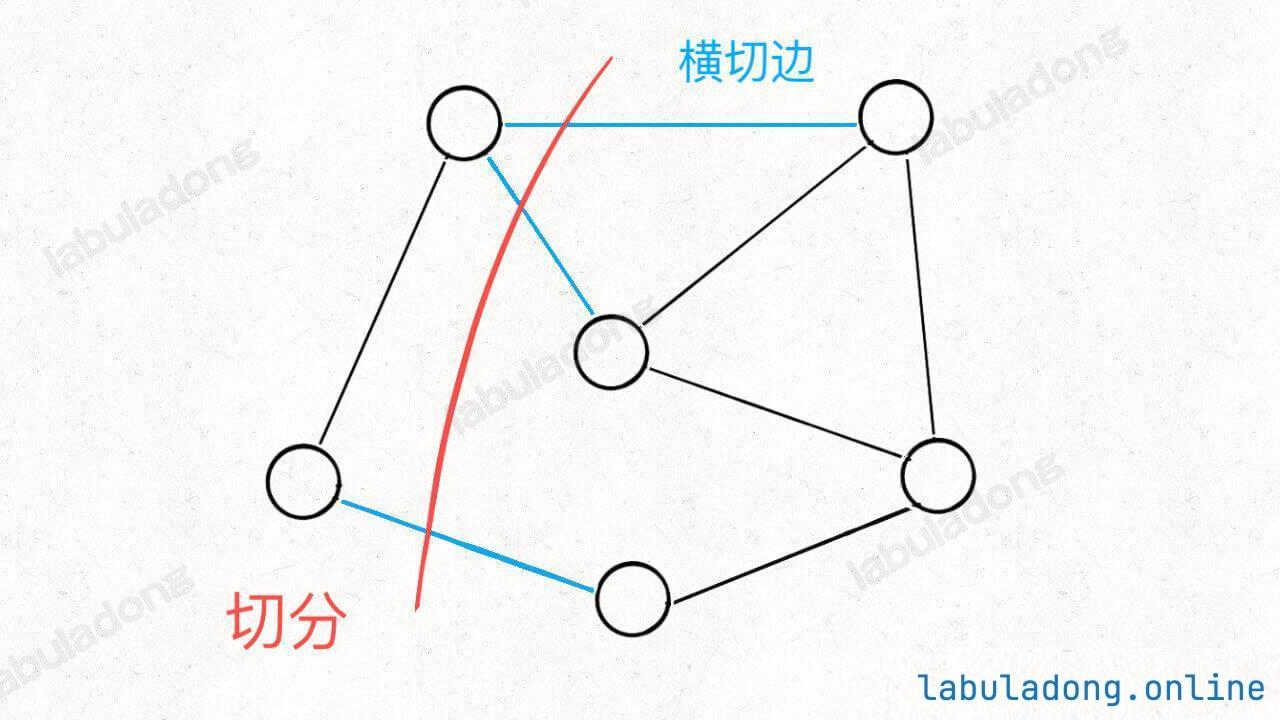Note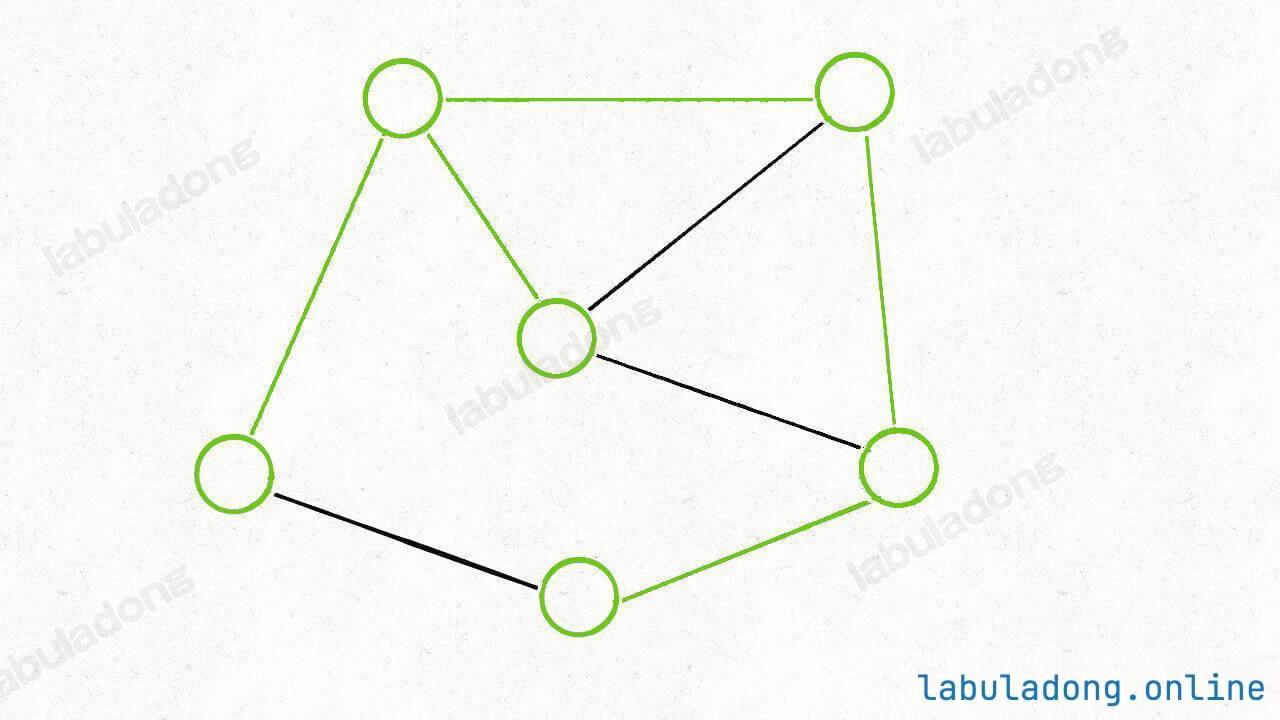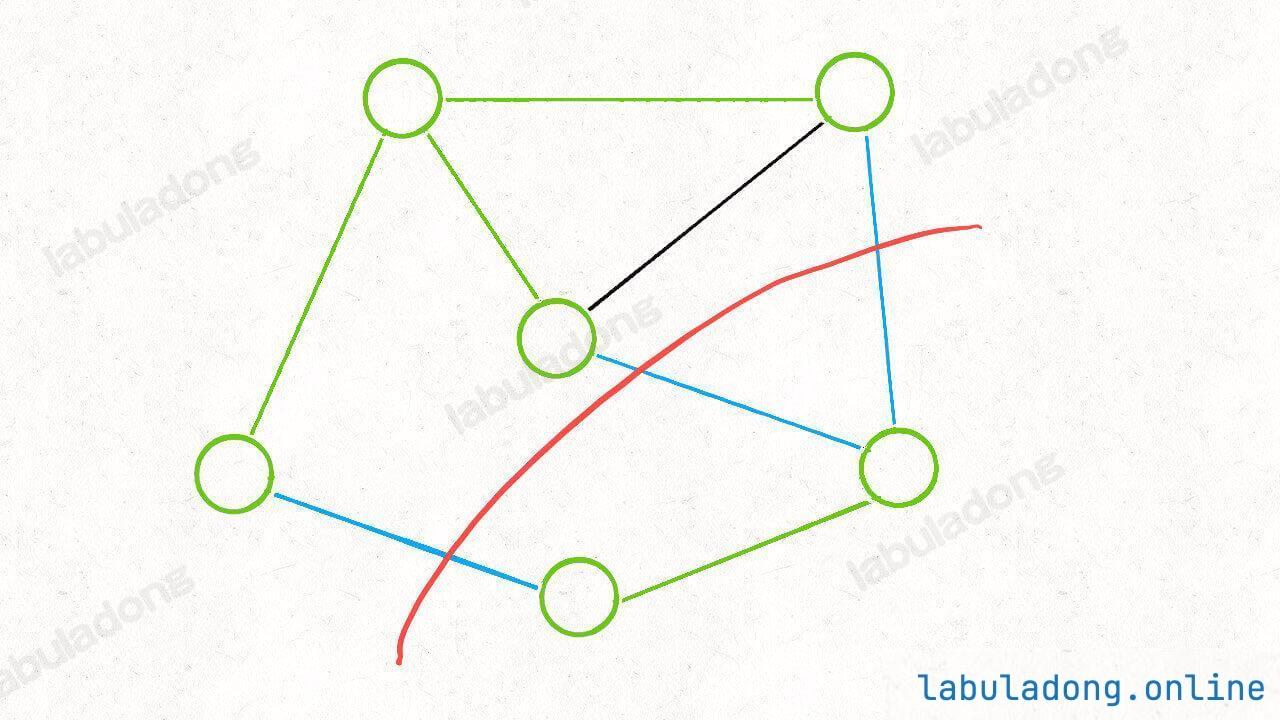### # Prim 算法实现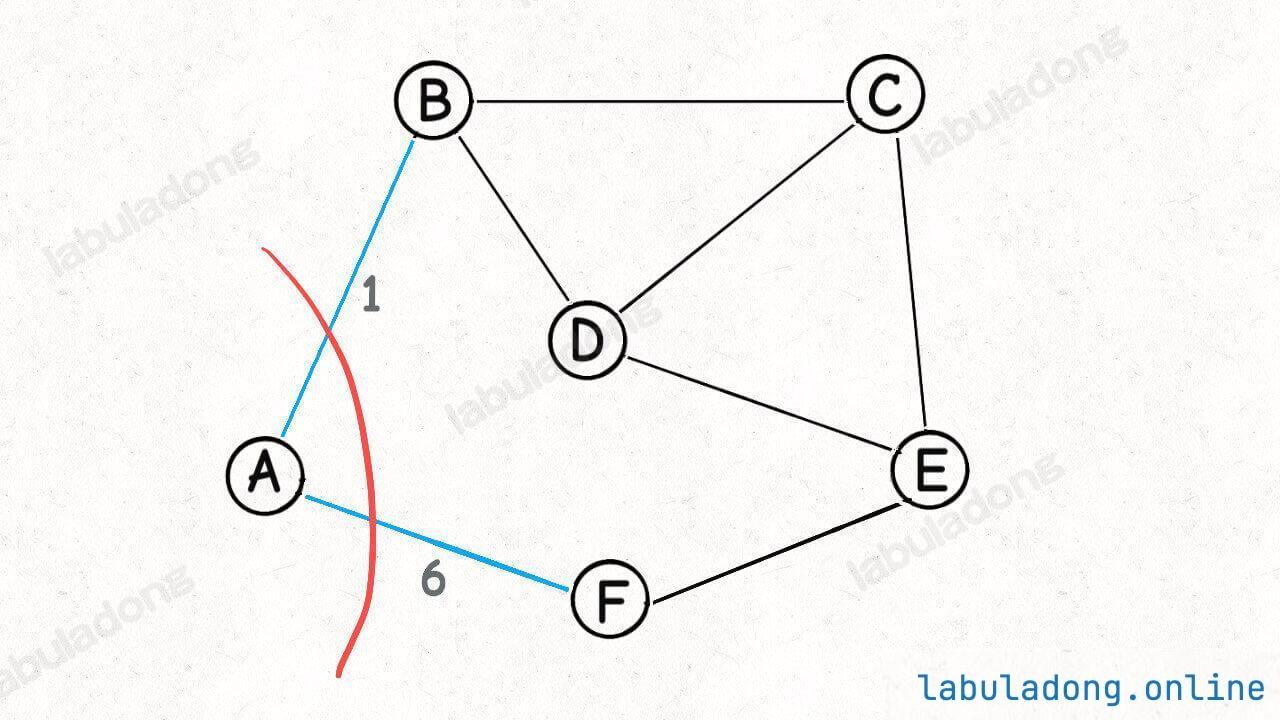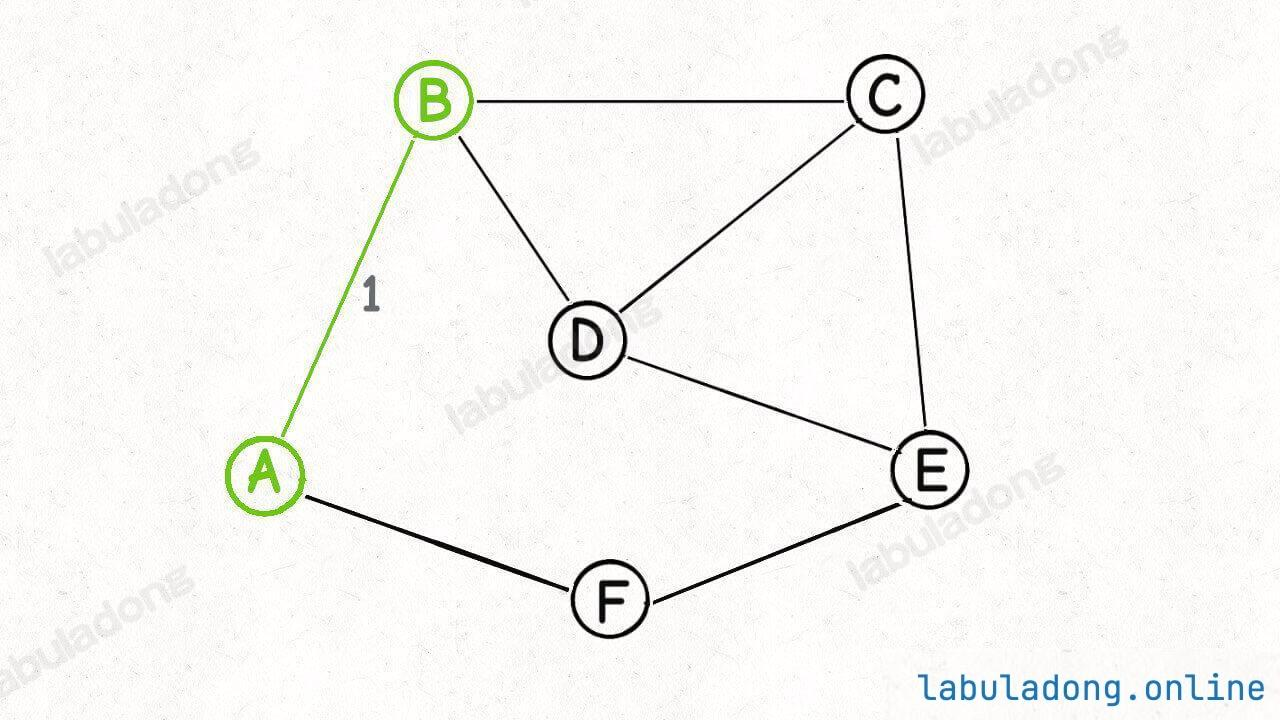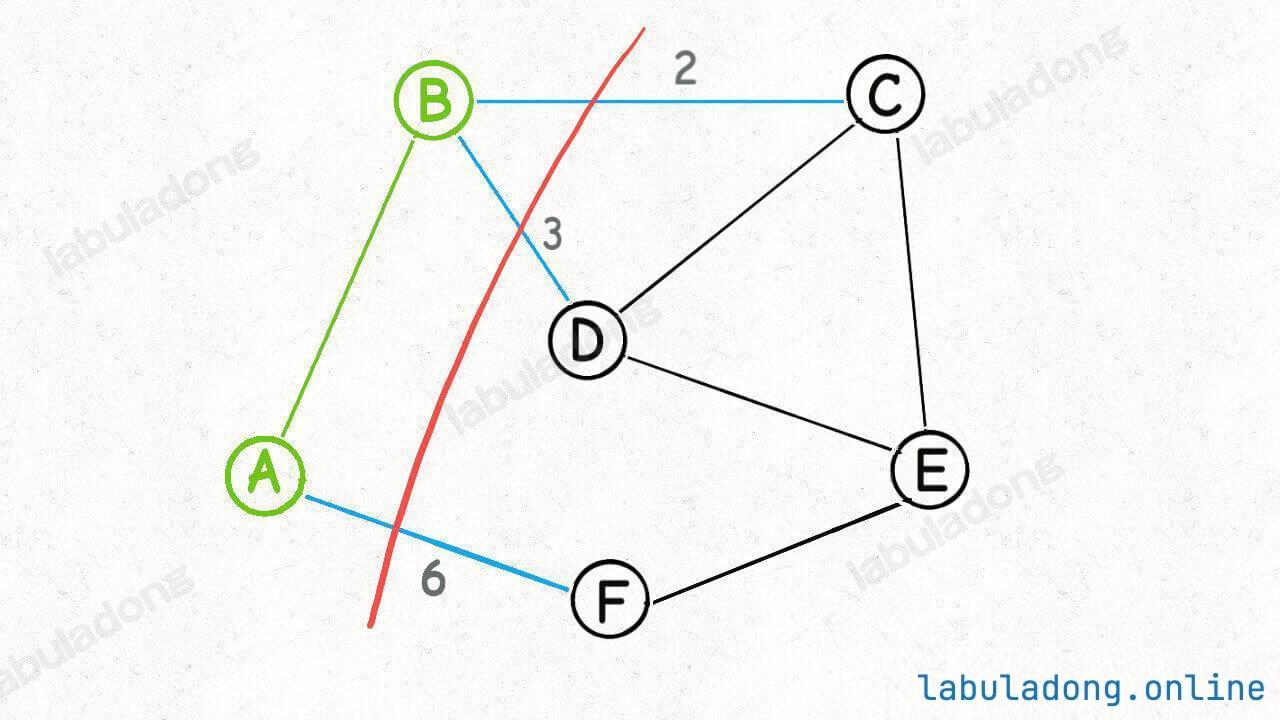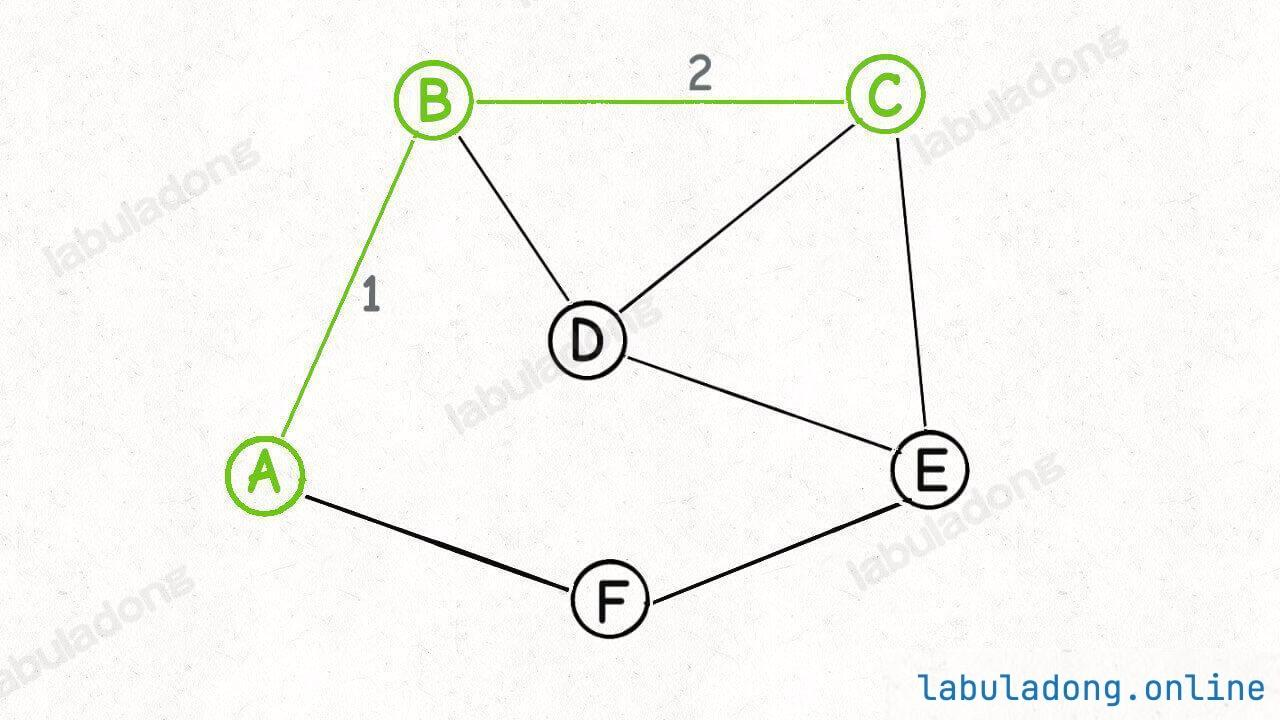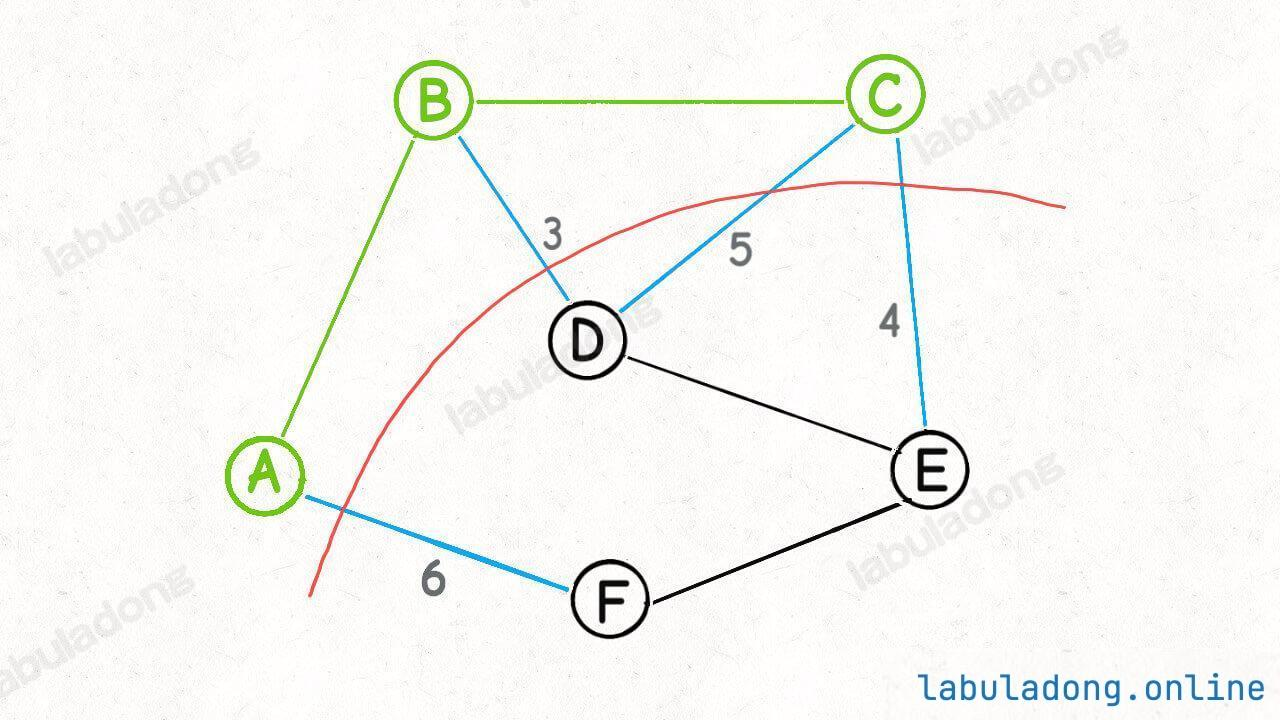Prim 算法的逻辑就是这样，每次切分都能找到最小生成树的一条边，然后又可以进行新一轮切分，直到找到最小生成树的所有边为止``````cut({A, B, C}) = cut({A, B}) + cut({C})
``````

`cut({C})` 就是节点 `C` 的所有邻边：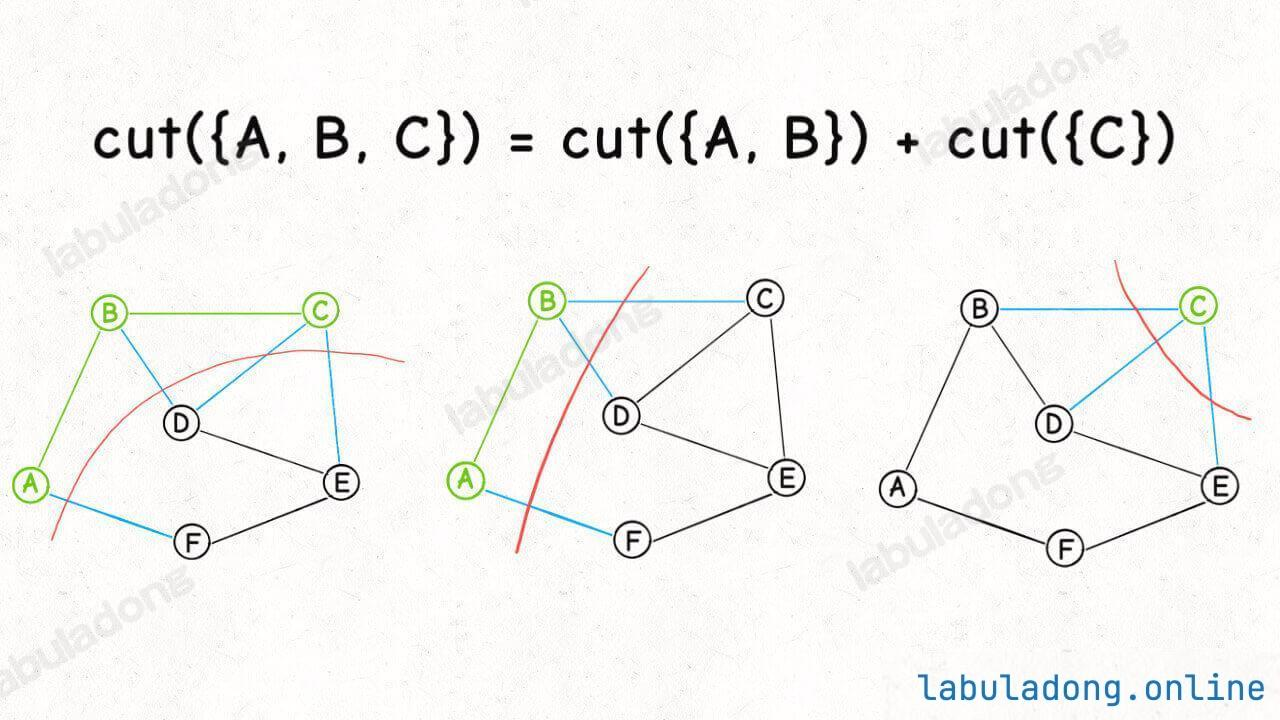``````class Prim {
// 核心数据结构，存储「横切边」的优先级队列
private PriorityQueue<int[]> pq;
// 类似 visited 数组的作用，记录哪些节点已经成为最小生成树的一部分
private boolean[] inMST;
// 记录最小生成树的权重和
private int weightSum = 0;
// graph 是用邻接表表示的一幅图，
// graph[s] 记录节点 s 所有相邻的边，
// 三元组 int[]{from, to, weight} 表示一条边
private List<int[]>[] graph;

public Prim(List<int[]>[] graph) {
this.graph = graph;
this.pq = new PriorityQueue<>((a, b) -> {
// 按照边的权重从小到大排序
return a - b;
});
// 图中有 n 个节点
int n = graph.length;
this.inMST = new boolean[n];

// 随便从一个点开始切分都可以，我们不妨从节点 0 开始
inMST = true;
cut(0);/**<extend down -100><div class="img-content"><img src="/algo/images/prim/4.jpeg" class="myimage"/></div> */
// 不断进行切分，向最小生成树中添加边
while (!pq.isEmpty()) {
int[] edge = pq.poll();/**<extend down -100><div class="img-content"><img src="/algo/images/prim/5.jpeg" class="myimage"/></div> */
int to = edge;
int weight = edge;
if (inMST[to]) {
// 节点 to 已经在最小生成树中，跳过
// 否则这条边会产生环
continue;
}
// 将边 edge 加入最小生成树
weightSum += weight;
inMST[to] = true;
// 节点 to 加入后，进行新一轮切分，会产生更多横切边
cut(to);/**<extend up -150><div class="img-content"><img src="/algo/images/prim/9.jpeg" class="myimage"/></div> */
}
}

// 将 s 的横切边加入优先队列
private void cut(int s) {
// 遍历 s 的邻边
for (int[] edge : graph[s]) {
int to = edge;
if (inMST[to]) {
// 相邻接点 to 已经在最小生成树中，跳过
// 否则这条边会产生环
continue;
}
// 加入横切边队列
pq.offer(edge);
}
}

// 最小生成树的权重和
public int weightSum() {
return weightSum;
}

// 判断最小生成树是否包含图中的所有节点
public boolean allConnected() {
for (int i = 0; i < inMST.length; i++) {
if (!inMST[i]) {
return false;
}
}
return true;
}
}
``````

Kruskal 算法是在一开始的时候就把所有的边排序，然后从权重最小的边开始挑选属于最小生成树的边，组建最小生成树。

Prim 算法是从一个起点的切分（一组横切边）开始执行类似 BFS 算法的逻辑，借助切分定理和优先级队列动态排序的特性，从这个起点「生长」出一棵最小生成树。

### # 题目实践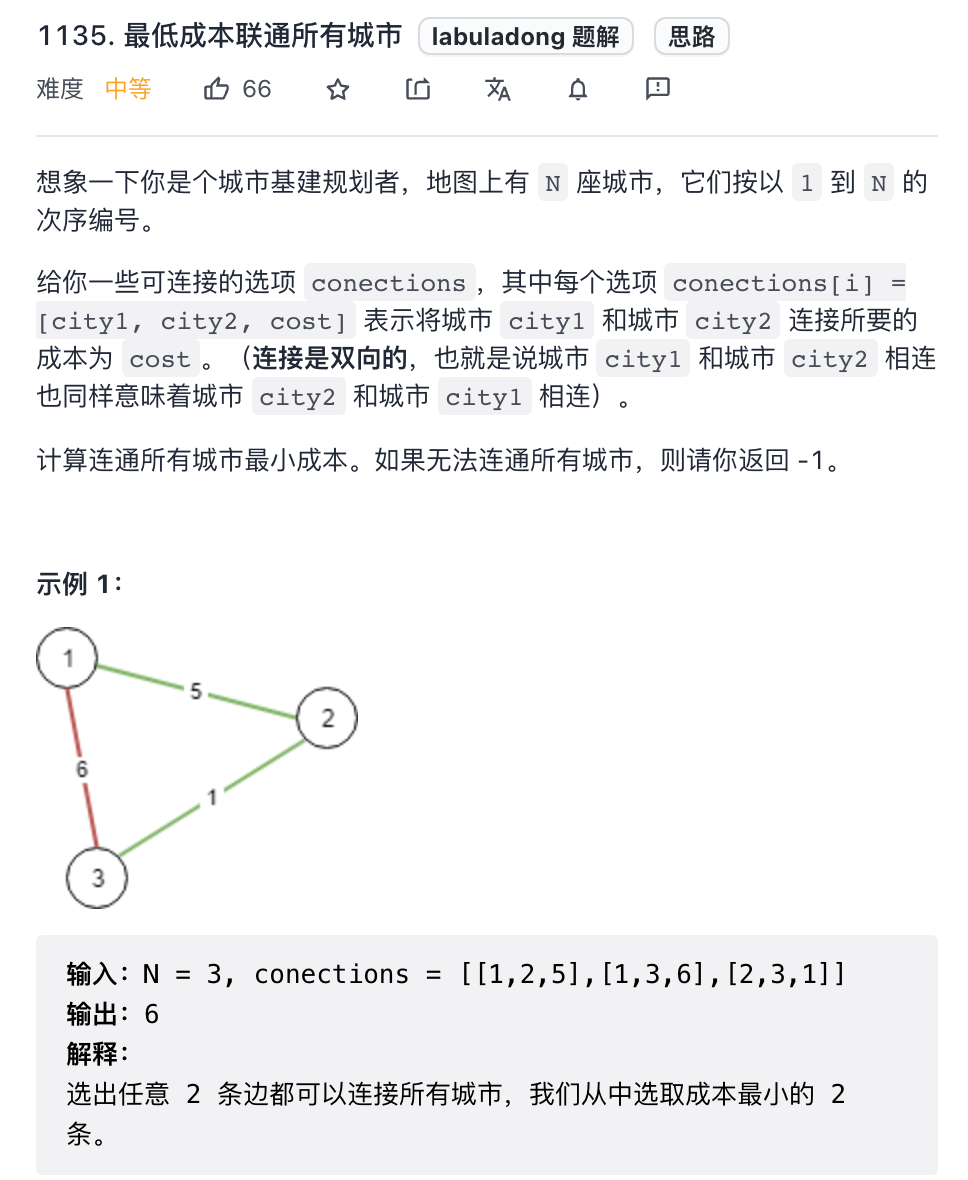``````int minimumCost(int n, int[][] connections);
``````

``````public int minimumCost(int n, int[][] connections) {
// 转化成无向图邻接表的形式
List<int[]>[] graph = buildGraph(n, connections);
// 执行 Prim 算法
Prim prim = new Prim(graph);

if (!prim.allConnected()) {
// 最小生成树无法覆盖所有节点
return -1;
}

return prim.weightSum();
}

List<int[]>[] buildGraph(int n, int[][] connections) {
// 图中共有 n 个节点
for (int i = 0; i < n; i++) {
}
for (int[] conn : connections) {
// 题目给的节点编号是从 1 开始的，
// 但我们实现的 Prim 算法需要从 0 开始编号
int u = conn - 1;
int v = conn - 1;
int weight = conn;
// 「无向图」其实就是「双向图」
// 一条边表示为 int[]{from, to, weight}
}
return graph;
}

class Prim { /* 见上文 */ }
``````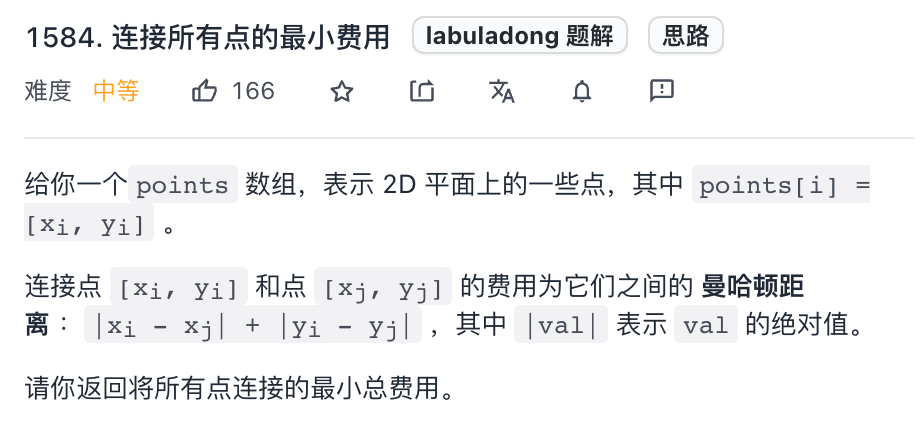``````points = [[0,0],[2,2],[3,10],[5,2],[7,0]]
````````````int minCostConnectPoints(int[][] points);
``````

``````public int minCostConnectPoints(int[][] points) {
int n = points.length;
List<int[]>[] graph = buildGraph(n, points);
return new Prim(graph).weightSum();
}

// 构造无向图
List<int[]>[] buildGraph(int n, int[][] points) {
for (int i = 0; i < n; i++) {
}
// 生成所有边及权重
for (int i = 0; i < n; i++) {
for (int j = i + 1; j < n; j++) {
int xi = points[i], yi = points[i];
int xj = points[j], yj = points[j];
int weight = Math.abs(xi - xj) + Math.abs(yi - yj);
// 用 points 中的索引表示坐标点
}
}
return graph;
}

class Prim { /* 见上文 */ }
``````